Disclaimer: This is an example of a student written essay.

Any scientific information contained within this essay should not be treated as fact, this content is to be used for educational purposes only and may contain factual inaccuracies or be out of date.

# Semi Empirical Formula For Neutrinoless Double Beta Decay

 ✅ Paper Type: Free Essay ✅ Subject: Physics ✅ Wordcount: 4980 words ✅ Published: 9th Mar 2018

Abstract

A Semi empirical formula for both phase space factor and Nuclear Matrix Element (NME) is developed for neutrinoless double beta decay, and the formula is used to compute the neutrinoless double beta decay half lives. The computed half lives for neutrinoless double beta decay are compared with the corresponding experimental values and with those predicted by QRPA model. The semi empirical formula predictions are found to be in good agreement with experimental data. The semi empirical formula is used to predict neutrinoless double beta decay of various isotopes Ca, Ge, Se, Zr, Mo, Pd, Cd, Sn, Te, Xe, Nd and Sm that exhibiting single beta decay. As our semi empirical formula predictions agree with the experimental data we hope that the present work will be useful for the future experiments.

Keyword: Neutrinoless double beta decay, Nuclear Matrix Element

1. Introduction

Double beta decay is a radioactive decay process where a nucleus releases two beta rays as a single process. Here two neutrons in the nucleus are converted in to two protons and in the process two electrons and two electron antineutrinos are emitted. In order for beta decay to be possible the final nucleus must have larger binding energy than the original nucleus. Double beta decay is difficult to study in most practically interesting cases, because both beta decay and double beta decay are possible, with probability favouring beta decay. The double beta decay is usually studied only for beta stable nuclei. Like single beta decay, double beta decay does not change the mass number A. More than 60 naturally occurring isotopes are capable of undergoing double beta decay.

Double beta decay is of two types; the two neutrino and neutrinoless double beta decay. The two neutrino double beta decay [2β(2ν)]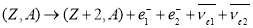which involves the transformation of two neutrons into two protons conserves not only the electric charge but also the lepton number. On the other hand neutrinoless double beta decay [2β(0ν)]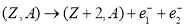violates lepton number conservation and is therefore forbidden in the standard electroweak theory. According to this theory neutrinos are massless. The observation of neutrino mass and oscillation is a clear example of a phenomenon at variance with the standard model.

There are different models for explaining the double beta decay process. Among them, two methods are mainly used to calculate Nuclear Matrix Elements (NME) for 2β(0ν) decays. One is the family of Quasi particle Random Phase Approximation (QRPA) . This method has been used by different groups and varieties of techniques are employed with results for most of the possible emitters . The other method concerned to double beta decay process is the interacting shell model (ISM) . It has been shown that as the difference in deformation between parent and daughter grows, the NME’s of both the neutrinoless and two neutrino mode decreases rapidly.

If you need assistance with writing your essay, our professional essay writing service is here to help!

The interest in double beta decay spans more than six decades. In 1937 Racah  following the fundamental suggestion of Majorana , discussed the possibility of a neutrinoless transformation of two neutrons into two protons plus two electrons. Even earlier Geoppert-Mayer  evaluated the decay rate of 2β(2ν) mode and realized that the corresponding half lives could exceed 1020years. Furry  shortly afterwards estimated that 2β(0ν) should be much faster than 2β(2ν) decay. Thus the stage was set for the realization that observation of the 2β(0ν) decay would establish that the neutrino is a massive Majorana particle. In 1982 J. Schechter-Valle while regarding 2β(0ν) decay suggested the existence of Majorana mass of the neutrino in the frame work of Gauge theories . In 1984 Fiorini et al  introduced a program to develop low temperature detectors for 2β decay search. Next year Doi et al  made a fundamental theoretical analysis of 2β decay to obtain the main formulae for probability of decay, energy and angular electron spectra. In 1986 using QRPA model Vogel et al  gave satisfactory agreement between theoretical and experimental 2β(2ν) half life values.

Neutrinoless double beta decay is of great interest for studying the fundamental properties of neutrino beyond the standard electro-weak theory. High sensitivity 2β(0ν) studies are the unique and practical ways for studying the Majorana nature of neutrinos, the neutrino mass spectrum, the absolute neutrino mass scale, the majorana CP phases and other fundamental properties of neutrinos in the foreseeable future. The first experiment  to claim 2β(0ν) is the Klapdor, HM experiment done in the year 2001. Numerous experiments like COBRA, GERDA etc have been carried out to search neutrinoless double beta decay and 48Ca, 76Ge, 82Se, 96Zr, 100Mo, 116Cd, 128Te, 150Nd, 238U are some of the isotopes exhibiting neutrinoless double beta decay [13-17].

For the double beta decay processes, two crucial ingredients are the phase space factors and the Nuclear Matrix Elements (NME). A general theory of phase space factors was developed by Doi et al. [18, 19] following the previous work of Primakoff and Rosen , and Konopinski . It was reformulated by Tomoda  by approximating the electron wave functions at the nuclear radius and without inclusion of electron screening. The Nuclear Matrix Element depends on the nuclear structure of the nuclei involved in the decay. The expression for Nuclear Matrix Element can be written in general as the sum of three components  as(1)

Where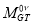,,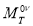, are the Gamow-Teller, Fermi and tensor components respectively.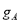is the axial vector coupling constant and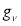is the vector coupling constant.

The present work aims to develop a semi empirical formula for both phase space factor and Nuclear Matrix Element for computing the neutrinoless double beta decay half life. By using this formula we would like to predict the possibility of 2β(0ν) decay from various isotopes exhibiting single beta decay. The details of the semi empirical formula are given in Section 2 and results, discussion and conclusion are given in Section 3.

1. The semi empirical formula

In the standard scenario, when 2β(0ν) decay process occurs by exchange of light Majorana neutrinos between two nucleons inside the nucleus, and in the presence of left handed weak interactions, the life time expression can be written as a product of three factors and is given as(2)

Where G is the phase space factor for this decay mode,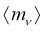is the effective neutrino mass parameter, me is the electron mass and M are the Nuclear Matrix Elements depending on the nuclear structure of the nuclei involved in the decay.

The phase space factor depends on the energy decay Qββ and nuclear charge Z and studied the dependence of phase space factor with ZQ3 and Z2Q6 for various isotopes undergoing neutrinoless double beta decay. From the observed dependence of phase space factor taken from ref , with ZQ3 and Z2Q6 we have developed a semi empirical formula for the phase space factor. Using ZQ3, Z2Q6 and Z3Q9 as variables, a new formula is obtained and is given as,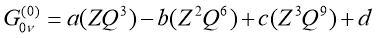(3)

The constants are,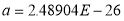,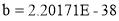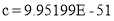,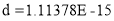Due to the two-body nature of the transition operator, the NMEs can also be expressed as a sum of product of two-body transition densities (TBTDs) and matrix elements of the two-body transition operators for two-particle states. We have studied the dependence of nuclear matrix element values taken from  with Z-1/3 for various isotopes undergoing neutrinoless double beta decay and a new formula is obtained by making least-squares fit to the nuclear matrix elements data and is given as,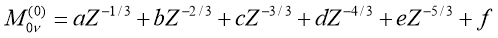(4)

The constants are,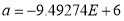,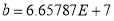,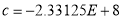,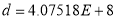,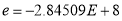,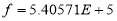The comparison of the computed nuclear matrix elements using the present formula with the values of Ref  and comparison of computed phase space factor with the values of Ref  are shown in Table 1.

1. Results, discussion and conclusion

The Q value for double beta decay of mother nuclide with mass mm to the daughter nuclide with mass md is given by the mass difference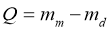which in turn can be written as a function of frequency ratio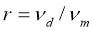and the electron mass me;(5)

In the present work Q values are computed using the experimental binding energies of Audi and Wapstra . The present empirical formula is applied for all the observed neutrinoless double beta decay isotopes. Column 7 of Table 1 represents the computed half-lives for neutrinoless double beta decay of various isotopes and is compared with the experimental values given in column 8 and QRPA values  in column 9. It is found from the table that our formula predictions are in good agreement with the experimental values and the QRPA values. The value of ν> is taken as 50meV and is obtained from Rodin et al .

We have applied the present formula for computing the phase space factor, Nuclear Matrix Element and half lives for various isotopes that exhibiting single beta decay. Tables 2 represents the computed Q values, Phase space factors, Nuclear Matrix Elements and half lives for neutrinoless double beta decay of various Ca, Ge, Se, Zr, Mo, Pd, Cd, Sn, Te, Xe, Nd and Sm isotopes. As our semi empirical formula prediction agree with the experimental data we hope that our prediction on neutrinoless double beta decay of various Ca, Ge, Se, Zr, Mo, Pd, Cd, Sn, Te, Xe, Nd and Sm isotopes will be a guide for future experiments.

­­­­­­­­­­­­­­­

Table 1. The computed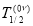,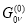and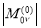for neutrino less double beta decay of various isotopes and their comparison with the experimental, QRPA and Ref  values

_____________________________________________________________________________________

 Isotope Q Value (MeV)x10-15 (y-1) _________________________(yrs) ______________________________ Present Ref. [ 25] Present Ref.  Present Expt. QRPA

_____________________________________________________________________________________

 48Ca 4.272 24.12 24.81 859.27 5.86e+21 >5.8E+22 76Ge 2.039 6.44 2.36 2.9 2.48 1.93e+27 >1.9E+25 2.49E+27 82Se 2.995 13.06 10.16 3.04 2.10 8.63e+26 >3.6E+23 8.13E+26 96Zr 3.35 22.59 20.58 3.92 0.40 3.01e+26 >9.2E+21 7.70E+27 100Mo 3.034 16.08 15.92 3.48 1.24 5.37e+26 >1.1E+24 1.45E+27 110Pd 2.018 7.91 4.82 2.57 2e+27 116Cd 2.814 14.73 16.7 2.37 1.31 1.26e+27 >1.7E+23 1.13E+27 124Sn 2.287 10.25 9.04 2.38 1.8e+27 128Te 0.866 1.93 0.59 2.53 1.47 8.47e+27 >1.5E+24 2.52E+28 130Te 2.527 12.38 14.22 2.53 1.36 1.32e+27 >2.8E+24 1.15E+27 136Xe 2.458 12.05 14.58 2.68 0.94 1.21e+27 >4.5E+23 2.18E+27 148Nd 1.929 8.54 10.1 1.37 6.54e+27 150Nd 3.371 62.85 63.03 1.37 1.96 8.88e+26 >1.8E+22 1.21E+26 154Sm 1.215 3.62 3.02 0.36 2.24e+29 160Gd 1.73 7.31 9.56 3.09 1.5e+27 1.30E+21 198Pt 1.047 3.17 7.56 66.26 7.5e+24

_____________________________________________________________________________________

Table 2. The computed Q values, Phase space factors, nuclear matrix elements and the predicted half lives for neutrino less double beta decay of various Ca, Ge, Se, Zr, Mo, Pd, Cd, Sn, Te, Xe, Nd and Sm, Gd and Pt isotopes

____________________________________________________________

 Isotope Q value (KeV)(y-1)T1/2 (yrs)

____________________________________________________________

 46Ca 988.3 1.58618E-15 859.272 8.92E+22 47Ca 2592.3 7.53405E-15 859.272 1.88E+22 49Ca 7269.8 3.40780E-12 859.272 4.15E+19 48Ca 4273.7 2.41768E-14 859.272 5.85E+21 50Ca 11855.7 3.44796E-10 859.272 4.10E+17 51Ca 13867.8 1.44900E-09 859.272 9.76E+16 52Ca 16955.0 9.01213E-09 859.272 1.57E+16 75Ge 312.6 1.13809E-15 2.90139 1.09E+28 76Ge 2039.1 6.44488E-15 2.90139 1.93E+27 77Ge 3385.6 1.71285E-14 2.90139 7.24E+26 78Ge 5164.1 5.34962E-13 2.90139 2.32E+25 79Ge 6427.6 4.72938E-12 2.90139 2.62E+24 80Ge 8244.9 5.07807E-11 2.90139 2.44E+23 81Ge 10089.5 3.30371E-10 2.90139 3.76E+22 82Se 2995.5 1.30683E-14 3.04409 8.63E+26 83Se 4640.7 2.21947E-13 3.04409 5.08E+25 84Se 6479.0 6.21762E-12 3.04409 1.81E+24 85Se 9052.0 1.46232E-10 3.04409 7.71E+22 86Se 12724.6 3.31456E-09 3.04409 3.40E+21 87Se 14129.4 8.57934E-09 3.04409 1.31E+21 88Se 15812.0 2.37729E-08 3.04409 4.74E+20 94Zr 1142.9 2.52372E-15 3.91925 2.69E+27 95Zr 2049.7 7.48180E-15 3.91925 9.09E+26 96Zr 3347.7 2.25176E-14 3.91925 3.02E+26 97Zr 4593.8 3.46849E-13 3.91925 1.96E+25 98Zr 6824.7 1.72152E-11 3.91925 3.95E+23 99Zr 8197.8 9.63615E-11 3.91925 7.06E+22 100Zr 9584.0 4.08099E-10 3.91925 1.67E+22 101Zr 10051.0 6.31459E-10 3.91925 1.08E+22 102Zr 11817.0 2.76762E-09 3.91925 2.46E+21 103Zr 12480.0 4.54642E-09 3.91925 1.50E+21 98Mo 112.3 1.11526E-15 3.47678 7.75E+27 99Mo 1651.2 5.10021E-15 3.47678 1.69E+27 100Mo 3035.0 1.60950E-14 3.47678 5.37E+26 101Mo 4438.7 2.88638E-13 3.47678 2.99E+25 102Mo 5541.0 2.68528E-12 3.47678 3.22E+24

_____________________________________________________________

Table 2. Continued…..

____________________________________________________________

 Isotope Q value (KeV)(y-1)T1/2 (yrs)

____________________________________________________________

103Mo

6408.8

1.10330E-11

3.47678

7.83E+23

104Mo

7759.0

6.71620E-11

3.47678

1.29E+23

105Mo

8588.0

1.72446E-10

3.47678

5.01E+22

106Mo

10067

7.43636E-10

3.47678

1.16E+22

107Mo

11430

2.37009E-09

3.47678

3.65E+21

109Pd

901.0

1.92669E-15

2.56879

8.22E+27

110Pd

2004.0

7.81588E-15

2.56879

2.03E+27

111Pd

3253.5

2.48567E-14

2.56879

6.37E+26

112Pd

4244.5

2.49383E-13

2.56879

6.35E+25

113Pd

5359.3

2.60657E-12

2.56879

6.07E+24

114Pd

6523.9

1.74643E-11

2.56879

9.06E+23

115Pd

7690.5

8.20373E-11

2.56879

1.93E+23

116Pd

8759.0

2.73683E-10

2.56879

5.78E+22

117Pd

9895.0

8.38279E-10

2.56879

1.89E+22

118Pd

11239

2.67934E-09

2.56879

5.91E+21

114Cd

540.1

1.30076E-15

2.37189

1.43E+28

115Cd

1945.5

7.60027E-15

2.37189

2.44E+27

116Cd

2809.1

1.46594E-14

2.37189

1.27E+27

117Cd

3975.0

1.46594E-14

2.37189

1.25E+26

118Cd

4947.1

1.48733E-13

2.37189

1.37E+25

119Cd

6158.4

1.35555E-12

2.37189

1.61E+24

120Cd

7131.1

1.15360E-11

2.37189

4.01E+23

121Cd

8144.1

4.62494E-11

2.37189

1.17E+23

122Cd

9215.9

1.59314E-10

2.37189

3.73E+22

123Cd

10510.5

4.97662E-10

2.37189

1.12E+22

124Cd

11526.8

1.65584E-09

2.37189

4.84E+2

View all

## DMCA / Removal Request

If you are the original writer of this essay and no longer wish to have your work published on UKEssays.com then please:

Related Services

Prices from

KD47

Approximate costs for:

• 1000 words
• 7 day deliveryHumanity University

Dedicated to your worth and value as a human being!

Related LecturesFreelance Writing Jobs

Looking for a flexible role?
Do you have a 2:1 degree or higher?

Study Resources

Free resources to assist you with your university studies!We can help with your essay

Part of Business Bliss Consultants FZEUseful Resources